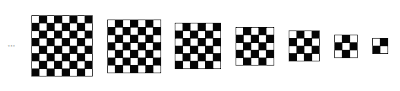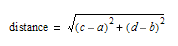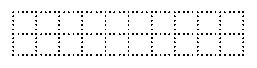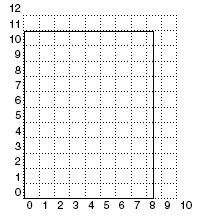## Wednesday, September 19, 2007

### Math Without Math: Distances

I'll go back to rasters and vectors, but I wanted to talk about one more idea on Cartesian coordinates, basically, on thinking of a sheet of paper (or any surface) as a grid of rows and columns.

If we make the rows and columns the same size, like inches or centimeters, then we can measure distances just by knowing where two points are. The simple case is if you have two points that are on the same horizontal or vertical line. For example, suppose two points are both 1 inch from the bottom of a page. One point is 2 inches from the left edge, and the other is 7 inches from the left edge. You can write this as (2,1) and (7,1), meaning two inches from the left and one inch from the bottom, or seven inches from the left and one inch from the bottom. Then the distance between them is 7 - 2, or 5 inches. (C’mon, you can handle a little subtraction, can't you?)

The harder case is if the two points are not on a horizontal or vertical line. Then you need a little bit of math called the Pythagorean Theorem. If one point is 4 inches from the left and 7 inches from the bottom (i.e., (4, 7)), and the other is 7 inches from the left and 3 inches from the bottom (7, 3), then the distance is five inches. How do we know that? Here are two methods:

The math way ...The non-math way ...
Get a ruler.

But if you want the gritty details, which would entitle you to say Pythagorean Theorem to your friends, you need to know what a square root is. The square of a number is that number multiplied by itself. So 25 is the square of 5 (i.e., 5 × 5), 16 is the square of 4 (4 × 4), etc. The square root is the reverse of that. It's the number which, when multiplied by itself, equals the original number. The square root of 25 is 5, the square root of 16 is 4, etc.

One way to think of this is to imagine you have a checkerboard with 64 squares in it. Then each side of the checkerboard would be 8 squares long. So 8 squared is 64, and the square root of 64 is 8. Likewise for a 7 × 7 (49 square) checkerboard, and for 9 × 9 (81 squares), 5 × 5 (25 squares), and so on for larger numbers and for smaller numbers.The Pythagorean Theorem says that to find the distance between two points, first find the horizontal and vertical distances, square them, add the results, and then take the square root of that. So if the two points were (a, b) and (c, d), then the distance would beNow I know that looks a lot like math, so let's unravel it to see what it's really saying. Let's look at the picture to start.Basically, what Pythagoras noticed was that if you make a square from each side of a right triangle, the two smaller squares add up to the larger square. In our example, we're trying to find the distance between two points. The first point is the top corner of the triangle, at (6, 8). (Just count the boxes, starting at the lower left corner. The second point is the bottom right corner of the triangle, at (9, 4). So the length of the bottom side of the triangle is 3 (which is 9-6), and the left side is 4 (8-4). However, it's not so easy to find the length of the third side, which is the distance we want. You can't just count the boxes.

If you square those lengths, you get 32=9 for the bottom side, and 42=16 for the left side. Adding 9+16=25, which happens to be 52. So the length of the long diagonal side is 5.

I purposely picked a triangle with sides 3, 4 and 5 to make the numbers come out simple. I could, for example, have used 4 and 7 for the short sides, but then the diagonal would be 8.0622577.... The Pythagorean Theorem holds for any right triangle. A right triangle is a triangle that has one corner which is a right, or square, angle. You can see in the diagram above the bottom left corner is a right angle.

If you want some math jargon to sling around, a corner of a triangle is called a vertex, and the long side of a right triangle is called the hypotenuse. Remember that scene in The Wizard of Oz where the Scarecrow gets a diploma and suddenly realizes that "the square of the hypotenuse is equal to the sum of the squares of the other two sides?" That's the Pythagorean Theorem. Now you too can be a Doctor of Thinkology!

In math, a theorem is an idea that seems to be true, but hasn't been proven. So the Pythagorean Theorem hasn't had a rigorous mathematical proof yet. However, keep in mind that Pythagoras came up with this idea over 2500 years ago, and no one has found a single case where it doesn't work, so it's pretty safe.

## Wednesday, September 12, 2007

### Rasters and Vectors

I want to take a side trip from the "Math Without Math" discussion to talk about rasters and vectors. Since time immemorial (actually, probably early 1960's or so), these have been the two predominant ways of describing images displayed on the computer. Even today, most 2D graphics software falls into one or the other of these categories:

Raster
• Photoshop
• Painter
• Whatever came with your digital camera
Vector
• Illustrator
• InDesign
• Uh ... something else

So what's really going on here? The difference has to do with the way the software thinks about the picture it's working on. Raster software thinks the picture is just a bunch of colored dots, arranged in rows and columns, to make up a picture. It's kind of like a tile mosaic, or like one of those weird paintings by Seurat of people in very formal clothes trying to relax while they disintegrate into blobs of paint. There's no structure to the picture. The software knows nothing about which colored dots make up a face, and which ones make up a rock or a tree or anything else. There are just lots of dots.

Those mosaics of colored dots are called rasters, or sometimes bitmaps. Raster is an old term that comes from TV and video electronics, and describes how an old tube TV displays pictures by sweeping a beam of electrons across the glass screen. The electrons would hit dots of phosphor on the back of the glass, causing them to light up. Because the beams would sweep from side to side, top to bottom, they covered the whole screen, though the intensity of the beam would change, making some of the phosphor dots glow brighter than others. That's how a picture got drawn on a TV screen, and that's why pictures made up of dots of colors are called rasters. Each individual dot is called a pixel, short for "picture element."

As the picture below shows, the electron beam of a raster scan display sweeps back and forth over the screen in a kind of zig-zag pattern.In each horizontal row, there would be some number of phosphor dots that could be made to glow brighter or dimmer, based on how intense the beam is when it hits that dot. The beam would swing left to right (seen from our side of the screen), then shut off and swing back to the left and down one row to begin the next row. In this way, every dot on the screen would get hit.

There was another kind of display screen called a vector display. In a vector display, the electron beam wouldn't sweep over the entire screen row by row. Instead, it would just go from point to point, according to the electronic signal it got. So it could draw a smooth line from one corner of the screen to the other, or draw a circle in the middle, or whatever. The lines and curves weren't made up of dots in the way the raster displays were. Instead, each line and curve was more or less a smooth, continuous shape, drawn by the electron beam.

There were advantages to each kind of display. The raster display could easily be adapted to show color, by using red, green and blue phosphor dots, and making them glow at different intensities to create various color mixes. (The dots were so close together that a person looking at the screen would basically see colors, rather than separate red, green and blue dots.) Also, because the raster display was painting every point on the screen, it was possible to display very photographic looking images like ... well, like TV.

Vector displays were somewhat more limited, but the quality of the lines and curves they could draw was magnificent, because they didn't have to draw a set of dots to look like a line, or a set of dots to look like a circle.

So a raster display might look like this:but up close, you can see that it's just made up of colored dots ...With a vector display, on the other hand, the quality is preserved, no matter how close you look, or how much you magnify the display:Another way to think of this is that raster images store only the actual look of the image ... what colors go where, the same as taking a picture with a digital camera. Vector images, on the other hand, store the shapes that make up the image ... lines, circles, text, etc. In a sense, vector images are like instructions for drawing a picture:
• put a line from point a to point b
• draw a circle at point c
• put a rectangle with one corner at point d and one at point e
etc.This is a very important difference in terms of what kinds of editing operations you can do, and I'll talk more about this later.

## Monday, September 10, 2007

### Math Without Math: Functions

One of the cool things about Cartesian coordinates is that we can describe pictures with numbers. This means that we can use computers for what they do best ... computing ... in helping to create and display pictures. One category of mathematical tools that we use for this is functions. Functions also show up in programming, with a similar meaning, so they're good things to know about.

A function is basically a rule for combining one or more numbers to get another number. For example, we can think of the average temperature in a city as a function of the month. For a Northern climate, the high temperature will be highest in the Summer months, lowest in the Winter months, and in between in the Fall and Spring. There will be some wiggling around because temperatures don't follow a hard and fast rule.

We can use Cartesian coordinates to visualize a function. We have two numbers: the month of the year, and the average temperature for that month. Whenever we have two related numbers like this, we can use them to indicate points on a graph. For the temperature, the graph might look something like this:This is really two functions: the high average temperature and the low average temperature in New York City for each month.\footnote{Source: New York City: Average Temperatures (http://www.ny.com/general/weather.html) Both of them rise and fall with the seasons, but the high temperature is ... um, higher.

Squaring a number is another example of a function. We can make a graph of numbers and their squares. It looks like this:The reverse of this, the square root, is also a function we can graph:Notice that the square root function looks like the square function flipped over on it's side. That's because it is. Remember that if you have two numbers, and one is the square of the other, than the other is the square root of the first. So we're really just switching the horizontal and vertical directions of the graph.

Sometimes a function will take two numbers and give you a third. For example, if you scan a piece of art at some resolution, the size of the file is a function of the width and height of the art. Change either one of those and you change the file size.. This is called a multi-variate function, since there are two variables. The function is area = width x length.

Since there are three numbers, the two variables and the result, we can't graph this function in 2 dimensions. We need to use 3 dimensions. The graph would look something like this:Who says math is not visual?

So what the heck does this mean? Suppose your art is only 1 inch tall, and so many inches wide.Then making it an inch longer adds just one extra square inch. If we scan at 300 samples per inch, then 1 square inch is about 270,000 bytes. That's because each sample is 3 bytes, and one inch has 300 of those samples. That's 900 bytes just for a one pixel wide scan. But a square in is 300 pixels wide, so we multiply again: 3 x 300 x 300 = 270,000.

However, if the picture were 2 inches tallthen each inch wider adds 2 square inches. So that means that as the width increases, the 2 inch tall picture grows twice as fast as the one inch tall one. A 3 inch tall picturewould grow three times as fast. We can graph this like this:Here the red line is the function that gives the file size of a 1 inch tall picture for different widths of the picture. The blue line gives the same function for a 2 inch tall picture, and the green line is for a 3 inch tall picture. As you can imagine, we could keep on for a 4 inch tall picture, 5 inches, and so on, and the lines would just keep getting steeper. So the taller the picture is, the more effect changing the width has, and vice versa.

We could graph this in 3D like this:We made all the lines blue, but each blue line still represents one height of the scanned picture, at different widths. The first blue line, closest to the front, is for pictures 1 inch tall. At the left end, where the width is very small, the overall size of the scanned file will be small. Towards the right end of that line, the width is larger, so the size of the scanned image file will be larger. The line behind that is for 2 inch tall scans, so it's steeper. Behind that is 3 inches, etc. So the taller the picture is, the more a small change in width will affect it.

## Friday, September 7, 2007

### Math Without Math: Cartesian Coordinates

As I said before, math seems hard to a lot of people because it requires learning a new language ... a language of symbols and notations evolved over centuries. In fact, the American Mathematical Society has a specialized typesetting system for authors to use in writing mathematical papers, so they can get that notation correct. The symbols are important to mathematicians because they have very precise meanings, and everyone who understands that language will interpret the symbols in the same way. Luckily, we don't have to worry about this. We don't need such precise, unambiguous language. We can describe in plain English the ideas behind the mathematical notation.

So here we explain a lot of concepts taken from various fields of advanced math. A lot of this often gets lumped under the heading engineering math, because ... well, it's math that's used by engineers. When engineers study this, it's divided into various subfields and specializations. Here, we're just present some topics that come up in our work.

Some of this stuff will be familiar, because you've seen it in school. Other stuff you probably haven't seen before, but you'll be able to pick it up. The goal is not to make you a mathematician. The goal is to give you a visual understanding of some mathematical ideas that show up in computer graphics all the time.

Cartesian coordinates

When you describe a picture on a page, you might say something is in the upper left corner, or the lower right, etc. In other words, you can use two positions to name a location. On position says how far up or down the page, and one says how far to the left or right. You could think of a page as having areas like this:

 TopLeft TopCenter TopRight MiddleLeft MiddleCenter MiddleRight BottomLeft BottomCenter BottomRight

If we divide the page up even more, we can give a location more precisely. Trying to name each part of the page gets pretty messy though ... "the lower right corner of the middle center area."
To deal with that, we use numbers:You may think it's kind of weird to count rows from bottom to top, but that's the tradition in math. Blame it on a guy named Rene Descartes, who's the same guy who said "I think therefore I am," so he must have been pretty smart (or at least he thought he was.) This whole system of identifying locations by two numbers is called Cartesian coordinates after him, and each number is a coordinate. It's also the tradition to give the horizontal coordinate first, and then the vertical. So when you see something like (47,92), it means 47 units from the left, and 92 units from the bottom.

This ability to describe any location on the page by two numbers is very important in both art and math. A vast amount of computer graphics programming depends on this, and there are some cool math tricks that take advantage of this way of looking at space. We'll look at some of this stuff later.

## Thursday, September 6, 2007

### Introduction

I've spent the better part of my multi-decade career developing software for artists and designers. This includes vector graphics editing programs, electronic publishing software, digital prepress software, and multimedia authoring tools.

In my spare time, I'm also a designer/illustrator. In other words, I spend my evenings using the same kinds of software tools that I've been developing all day. I hope this gives me a much greater awareness of how to develop tools for artists, designers and other visual creators. At the very least, it gives me a somewhat uncommon perspective on the commonly used programs like Photoshop, Illustrator, InDesign, Flash, etc. I hope to share some of that with you.

Although I may occasionally get into details about this or that feature of this or that program, I hope to keep this more on the conceptual level. That way, whatever you might gain from reading this will help understand some whole class of programs and features in general, instead of just XXX version YYY.

Some of these insights would benefit from a little understanding of ... (gasp) ... math! (What? Artists learn math?) Someone I know once commented that math is so non-visual. In fact, nothing is further from the truth. Math is very visual. Math textbooks are full of illustrations to help students understand the concepts behind the weird shorthand that mathematicians use.

I'm going to try to sprinkle a little of that very intuitive, very visual math in here, without relying (too much) on the weird shorthand. I call it Math Without Math.